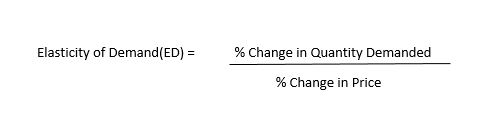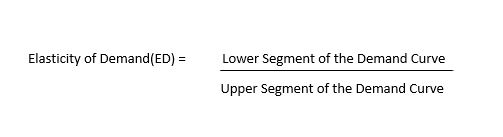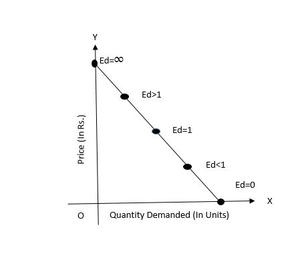# Price Elasticity of Demand

• Last Updated : 03 Jun, 2022

The degree of change (or the degree of extension or contraction in the demand curve) in response to a change in any economic factor related to the demand of a product is called Elasticity of Demand. The elasticity of demand is a measurement of the degree of change in demand in response to a given change in any factor which affects demand.

However, an important point here is that we always consider the percentage change in the values rather than considering the absolute value while calculating the elasticity of a commodity. Thus, we compare the percentage change in quantity demanded of a particular product to the percentage change in any other factor which affects demand (Price, Income, etc.).

### Types of Elasticity of Demand:

Elasticity of demand is further classified into three parts:-

1. Price Elasticity of Demand: Price Elasticity of Demand is defined as the measurement of percentage change in quantity demanded of a commodity in response to the percentage change in the own price of the commodity.

2. Cross Elasticity of Demand: Cross Elasticity of Demand is defined as the measurement of percentage change in quantity demanded of a commodity in response to the percentage change in the price of related goods (substitute or complementary goods). When the demand of a particular product fluctuates because of a change in the price of any other related product, it is called cross elasticity of demand. E.g. a fall in demand for cars because of the rise in the price of petrol. (Not in Syllabus)

3. Income Elasticity of Demand: Income Elasticity of Demand is defined as the measurement of percentage change in quantity demanded of a commodity in response to the percentage change in the income of the consumer.  When the demand for a particular product fluctuates because of a change in the aggregate income of the consumer, it is called income elasticity of demand. E.g. a rise in demand for cashews because of the rise in the income level of the consumer. (Not in Syllabus)

## Price Elasticity of Demand:

Consumers usually buy more when the price of the commodity falls and tends to buy less when the price of the commodity rises. Sometimes, with a greater change in the price of the product, the demand for that product does not change much and there can be a case where a slight change in the price of the commodity affects demand in a greater way. In Price Elasticity of Demand, the quantitative aspect of demand is studied. We will see the size of the movement of quantity demanded of a commodity related to its price.

The Price Elasticity of Demand for any goods measures the willingness of the consumers to buy less of the goods when the price rises and more of the goods when the price falls.

### Measurement of Price Elasticity of Demand: Two important methods of measuring Price Elasticity of Demand-

1. Proportionate or Percentage Method: This is the most popular method of measuring the price elasticity of demand. Under this method, Elasticity of Demand is measured by the ratio of the proportionate (percentage) change in quantity demanded to the proportionate change in the price of the commodity.Elasticity of Demand (Percentage Method)

2. Geometric Method: Geometric method measures the price elasticity of demand at different points on the demand curve. It is also called the ‘Point Method of measuring Elasticity of Demand’.Elasticity of Demand (Geometric Method)Point Method of measuring Elasticity of Demand

### Degrees or Types of Price Elasticity of Demand:

There are five distinct degrees or types by which various degree of elasticity is measured and graphically presented. We will see them one by one-

1. Perfectly Elastic Demand: A perfectly elastic demand refers to a situation where the demand is infinite at the prevailing price. It is a situation where the slightest rise in price causes the quantity demanded of the commodity to fall to zero.

2. Perfectly Inelastic Demand: A perfectly inelastic demand curve refers to a situation when a change in price causes no change in the quantity demanded. Even a great change in price does not impact the quantity demanded.

3. Unitary Elastic Demand: It refers to a situation when a change in quantity demanded in response to a change in own price of the commodity is such that total expenditure on the commodity remains constant.

4. Greater than Unitary Elastic Demand: Elasticity of Demand is greater than unitary elastic when a change in quantity demanded in response to a change in the price of the commodity is such that total expenditure on the commodity increases when the price decreases and total expenditure decreases when the price increases.

5. Less than Unitary Elastic Demand: Elasticity of Demand is less than unitary elastic when a change in quantity demanded in response to a change in the price of the commodity is such that the total expenditure on the commodity increases when the price rises and decreases when the price falls.

### Factors affecting Price Elasticity of Demand:

Price Elasticity of Demand depends on various factors. Some of the determinants of Price Elasticity of Demand are:

1. Nature of Commodity: There are different types of commodities prevailing in the market which affect the elasticity of demand. Ordinarily, necessaries like salt, oil, textbooks, etc., tend to have inelastic demand, whereas luxuries like air conditioners, fashionable garments, etc., have elastic demands.

2. Availability of Close Substitutes: Goods with close substitutes tend to have more elastic demand because it is easier for consumers to switch from that particular good to another. Whereas, goods with no close substitute tend to have inelastic demand because even if the price of that commodity rises, the consumers do not have a close substitute, and they have to buy that good only.

3. Diversity of Uses: Commodities that can be put to a variety of uses have elastic demand. On the other hand, if a commodity such as paper has only a few uses, its demand is likely to be less elastic.

4. Income Level of the Buyer: The Elasticity of Demand for goods also depends on the income level of its buyers. If the buyers of the goods are high-end consumers (with a high level of income), they will not be bothered by the rise in its price. So the elasticity of demand is expected to be low. On contrary, if the income level of the buyer is low, elasticity is expected to be high.

5. Time Horizon: Demand for any product is inelastic in a short period but elastic in long run. It is because in the long run, a consumer can change his consumption habits more conveniently than in the short period. It means that if the price of any product rises, then at that moment, the demand for that product is expected to be inelastic, but in some time the consumer will find a different approach to tackle this, and hence the demand is expected to become elastic.

My Personal Notes arrow_drop_up
Recommended Articles
Page :